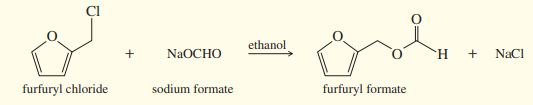×
Get Full Access to Organic Chemistry - 8 Edition - Chapter 6 - Problem 68sp
Get Full Access to Organic Chemistry - 8 Edition - Chapter 6 - Problem 68sp

×

# Furfuryl chloride can undergo substitution by both SN2 andISBN: 9780321768414 33

## Solution for problem 68SP Chapter 6

Organic Chemistry | 8th Edition

• Textbook Solutions
• 2901 Step-by-step solutions solved by professors and subject experts
• Get 24/7 help from StudySoup virtual teaching assistantsOrganic Chemistry | 8th Edition

4 5 1 341 Reviews
24
4
Problem 68SP

Furfuryl chloride can undergo substitution by both $$S_{N} 2 \text { and } S_{N} 1$$ mechanisms. Since it is a $$1^{\circ}$$ alkyl halide, we expect $$S_{N} 2$$ but not $$S_{N} 1$$ reactions. Draw a mechanism for the $$S_{N} 1$$ reaction shown below, with careful attention to the structure of the intermediate. How can this primary halide undergo $$S_{N} 1$$ reactions? Why is there no competition with $$E 2 \text { or } E 1$$ mechanisms?Equation Transcription:Text Transcription:

SN2 and SN1

SN2

SN1

SN1

SN1

E2 or E1

NaOCHO

NaCl

Step-by-Step Solution:

Solution 68SP :

Step 1:

Here, we have to draw a mechanism for the SN1 reaction shown below, and state how can this primary halide undergo SN1 reactions. And also mention why is there no competition with E2 or E1 mechanisms.Primary halides usually undergo SN2 reactions as they have less steric hindrance(it is prevention of chemical reaction by large groups present in a molecule) to the nucleophilic attack and also they are less stable.

Step 2 of 5

Step 3 of 5

##### ISBN: 9780321768414

This textbook survival guide was created for the textbook: Organic Chemistry, edition: 8. Organic Chemistry was written by and is associated to the ISBN: 9780321768414. The full step-by-step solution to problem: 68SP from chapter: 6 was answered by , our top Chemistry solution expert on 05/06/17, 06:41PM. Since the solution to 68SP from 6 chapter was answered, more than 1491 students have viewed the full step-by-step answer. This full solution covers the following key subjects: undergo, reactions, mechanisms, halide, Intermediate. This expansive textbook survival guide covers 25 chapters, and 1336 solutions. The answer to “?Furfuryl chloride can undergo substitution by both $$S_{N} 2 \text { and } S_{N} 1$$ mechanisms. Since it is a $$1^{\circ}$$ alkyl halide, we expect $$S_{N} 2$$ but not $$S_{N} 1$$ reactions. Draw a mechanism for the $$S_{N} 1$$ reaction shown below, with careful attention to the structure of the intermediate. How can this primary halide undergo $$S_{N} 1$$ reactions? Why is there no competition with $$E 2 \text { or } E 1$$ mechanisms? Equation Transcription:Text Transcription:SN2 and SN11°SN2SN1SN1SN1E2 or E1NaOCHONaCl” is broken down into a number of easy to follow steps, and 82 words.

## Discover and learn what students are asking

Calculus: Early Transcendental Functions : Inverse Trigonometric Functions: Integration
?In Exercises 1-20, find the indefinite integral. $$\int \frac{1}{\sqrt{1-(x+1)^{2}}} d x$$

Calculus: Early Transcendental Functions : Hyperbolic Functions
?In Exercises 7-14, verify the identity. $$\tanh ^{2} x+\operatorname{sech}^{2} x=1$$

Calculus: Early Transcendental Functions : Parametric Equations and Calculus
?In Exercises 1-4, find $$d y / d x$$. $$x=\sqrt{t}, y=4-t$$

Unlock Textbook Solution